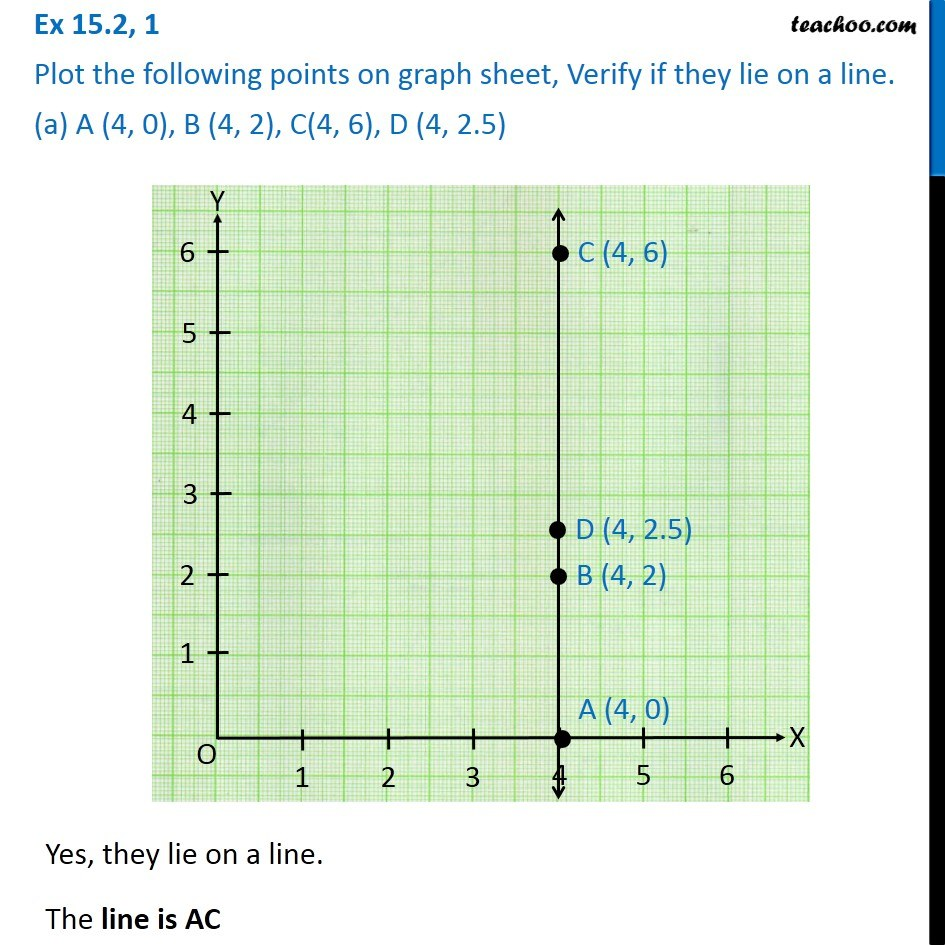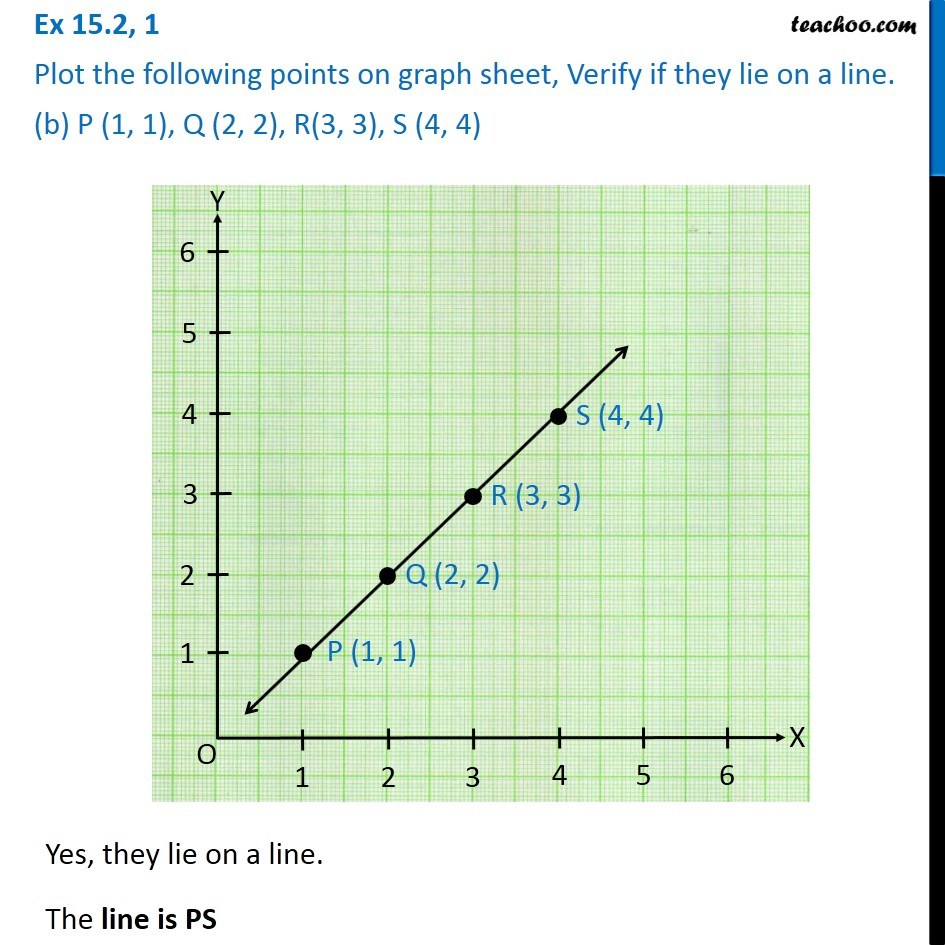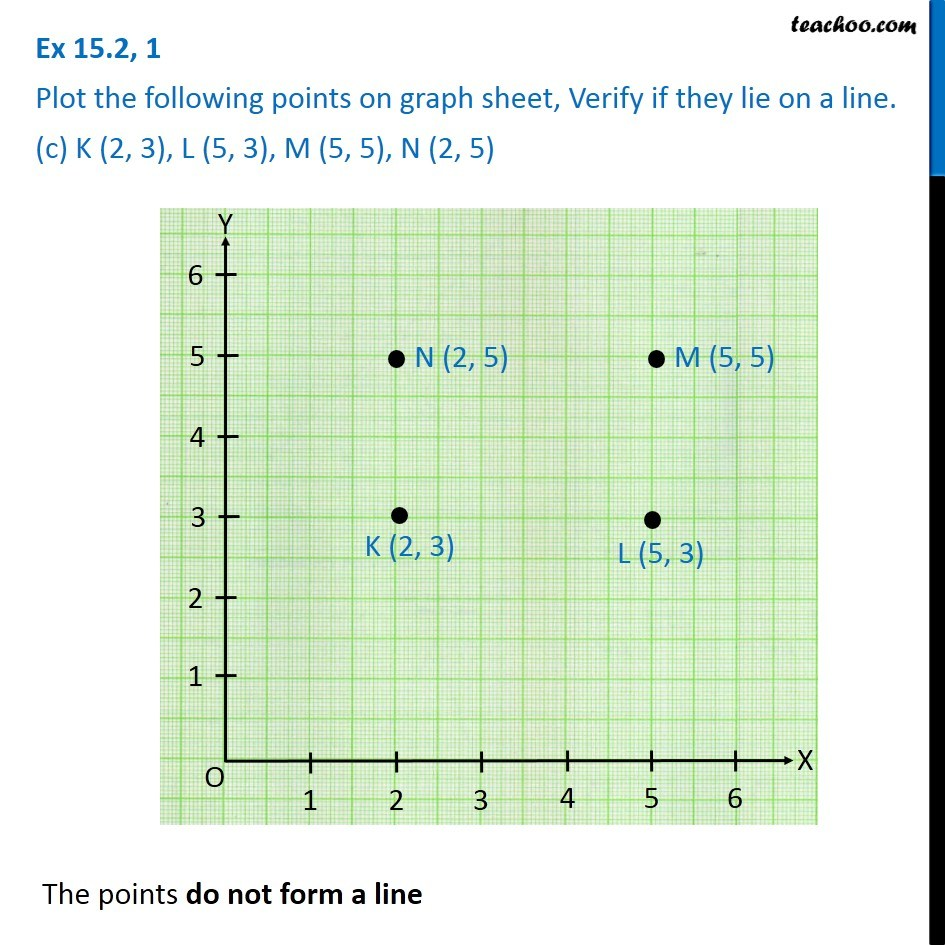Subscribe to our Youtube Channel - https://you.tube/teachoo

1. Chapter 15 Class 8 Introduction to Graphs
2. Serial order wise
3. Ex 15.2

Transcript

Ex 15.2, 1 Plot the following points on graph sheet, Verify if they lie on a line. (a) A (4, 0), B (4, 2), C(4, 6), D (4, 2.5)Yes, they lie on a line. The line is AC Ex 15.2, 1 Plot the following points on graph sheet, Verify if they lie on a line. (b) P (1, 1), Q (2, 2), R(3, 3), S (4, 4)Yes, they lie on a line. The line is PS Ex 15.2, 1 Plot the following points on graph sheet, Verify if they lie on a line. (c) K (2, 3), L (5, 3), M (5, 5), N (2, 5)The points do not form a line

Ex 15.2